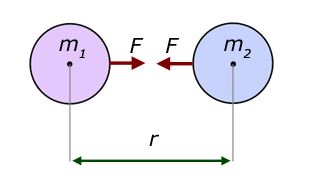# Newton's law of universal gravitation - Statement

Newton’s law of gravitation states that every particle in the universe attracts every other particle with a force which is directly proportional to the product of their masses and inversely proportional to the square of the distance between them.The mathematical representation of Newton’s law of gravitation is:

$$\begin{array}{l}F=-G\frac{m_{1}m_{2}}{r^{2}}\end{array}$$

Where,

• F is the force acting between the particles
• G is the gravitational constant
• m1 and m2 are the mass of the particles
• r is the distance between the particles

The SI unit of gravitational constant is N.m2.kg-2.

The CGS unit of gravitational constant is dyne.cm2g-2.

The dimensional formula of the gravitational constant is [M-1L3T-2].(188)(31)